• ## 向量的表示及协方差矩阵

万次阅读 多人点赞 2014-01-13 20:26:05
面对的数据被抽象为一组向量，那么有必要研究一些向量的数学性质。而这些数学性质将成为PCA的理论基础。向量运算：内积。两个维数相同的向量的内积被定义为： (a1,a2,⋯,an)T⋅(b1,b2,⋯,bn)T=a1b1+a2b2+⋯+anbn 内...
引言
当面对的数据被抽象为一组向量，那么有必要研究一些向量的数学性质。而这些数学性质将成为PCA的理论基础。
理论描述
向量运算即：内积。首先，定义两个维数相同的向量的内积为：

(a1,a2,⋯,an)T⋅(b1,b2,⋯,bn)T=a1b1+a2b2+⋯+anbn

内积运算将两个向量映射为一个实数。其计算方式非常容易理解，但是其意义并不明显。所以，我们分析内积的几何意义。假设A和B是两个n维向量，我们知道n维向量可以等价表示为n维空间中的一条从原点发射的有向线段，为了简单起见我们假设A和B均为二维向量，则

A=(x1,y1)
，

B=(x2,y2)
。则在二维平面上A和B可以用两条发自原点的有向线段表示，如图1所示。
现在，我们从A点向B所在直线引一条垂线。我们知道垂线与B的交点叫做A在B上的投影，再设A与B的夹角是a，则投影的矢量长度为

|A|cos(a)
，其中

|A|=x21+y21−−−−−−√
是向量A的模，也就是A线段的标量长度。注意这里我们专门区分了矢量长度和标量长度，标量长度总是大于等于0，值就是线段的长度；而矢量长度可能为负，其绝对值是线段长度，而符号取决于其方向与标准方向相同或相反。到这里还是看不出内积和这东西有什么关系，不过如果我们将内积表示为另一种我们熟悉的形式：

A⋅B=|A||B|cos(a)

现在事情似乎是有点眉目了：A与B的内积等于A到B的投影长度乘以B的模。再进一步，如果我们假设B的模为1，即让

|B|=1
，那么就变成了：

A⋅B=|A|cos(a)

也就是说，设向量B的模为1，则A与B的内积值等于A向B所在直线投影的矢量长度！这就是内积的一种几何解释，也是我们得到的第一个重要结论。在后面的推导中，将反复使用这个结论。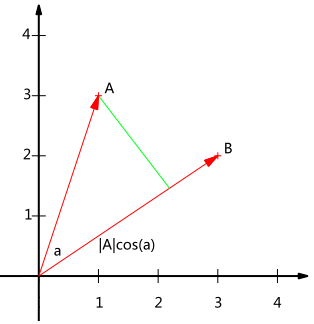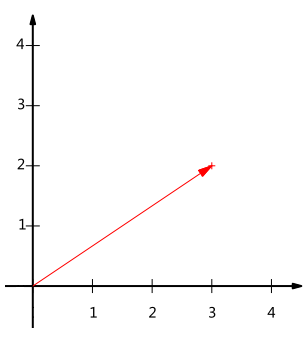图1                                                   　图2

基本节将继续在二维空间内讨论向量。上文说过，一个二维向量可以对应二维笛卡尔直角坐标系中从原点出发的一个有向线段。例如图2所示，这个向量，在代数表示方面，我们经常用线段终点的点坐标表示向量，例如上面的向量可以表示为(3,2)，这是我们再熟悉不过的向量表示。不过我们常常忽略，只有一个(3,2)本身是不能够精确表示一个向量的。我们仔细看一下，这里的3实际表示的是向量在x轴上的投影值是3，在y轴上的投影值是2。也就是说我们其实隐式引入了一个定义：以x轴和y轴上正方向长度为1的向量为标准。那么一个向量(3,2)实际是说在x轴投影为3而y轴的投影为2。注意投影是一个矢量，所以可以为负。更正式的说，向量(x,y)实际上表示线性组合：

x(1,0)T+y(0,1)T

不难证明所有二维向量都可以表示为这样的线性组合。此处(1,0)和(0,1)叫做二维空间中的一组基，如图3。所以，要准确描述向量，首先要确定一组基，然后给出在基所在的各个直线上的投影值，就可以了。只不过我们经常省略第一步，而默认以(1,0)和(0,1)为基。我们之所以默认选择(1,0)和(0,1)为基，当然是比较方便，因为它们分别是x和y轴正方向上的单位向量，因此就使得二维平面上点坐标和向量一一对应，非常方便。但实际上任何两个线性无关的二维向量都可以成为一组基，所谓线性无关在二维平面内可以直观认为是两个不在一条直线上的向量。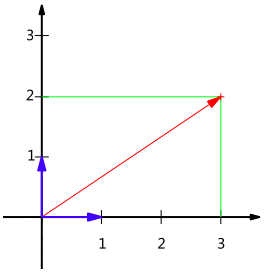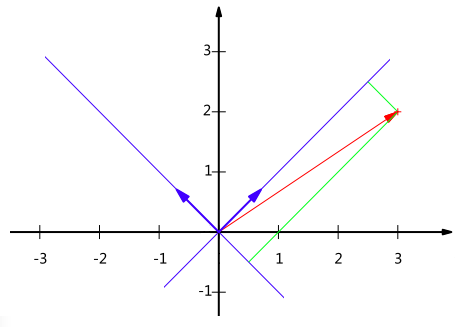图3                                                              图4
例如，(1,1)和(-1,1)也可以成为一组基。一般来说，我们希望基的模是1，因为从内积的意义可以看到，如果基的模是1，那么就可以方便的用向量点乘基而直接获得其在新基上的坐标了！实际上，对应任何一个向量我们总可以找到其同方向上模为1的向量，只要让两个分量分别除以模就好了。例如，上面的基可以变为

(12√,12√)
和

(−12√,12√)
。现在，我们想获得(3,2)在新基上的坐标，即在两个方向上的投影矢量值，那么根据内积的几何意义，我们只要分别计算(3,2)和两个基的内积，不难得到新的坐标为

(52√,−12√)
。图4给出了新的基以及(3,2)在新基上坐标值的示意图4所示。另外这里要注意的是，我们列举的例子中基是正交的（即内积为0，或直观说相互垂直），但可以成为一组基的唯一要求就是线性无关，非正交的基也是可以的。不过因为正交基有较好的性质，所以一般使用的基都是正交的。
基变换的矩阵表示
下面我们找一种简便的方式来表示基变换。还是拿上面的例子，想一下，将(3,2)变换为新基上的坐标，就是用(3,2)与第一个基做内积运算，作为第一个新的坐标分量，然后用(3,2)与第二个基做内积运算，作为第二个新坐标的分量。实际上，我们可以用矩阵相乘的形式简洁的表示这个变换：

(1/2√−1/2√1/2√1/2√)(32)=(5/2√−1/2√)

太漂亮了！其中矩阵的两行分别为两个基，乘以原向量，其结果刚好为新基的坐标。可以稍微推广一下，如果我们有m个二维向量，只要将二维向量按列排成一个两行m列矩阵，然后用“基矩阵”乘以这个矩阵，就得到了所有这些向量在新基下的值。例如(1,1)，(2,2)，(3,3)，想变换到刚才那组基上，则可以这样表示：

(1/2√−1/2√1/2√1/2√)(112233)=(2/2√04/2√06/2√0)

于是一组向量的基变换被干净的表示为矩阵的相乘。
一般的，如果我们有M个N维向量，想将其变换为由R个N维向量表示的新空间中，那么首先将R个基按行组成矩阵A，然后将向量按列组成矩阵B，那么两矩阵的乘积AB就是变换结果，其中AB的第m列为A中第m列变换后的结果。数学表示为：

⎛⎝⎜⎜⎜⎜p1p2⋮pR⎞⎠⎟⎟⎟⎟(a1a2⋯aM)=⎛⎝⎜⎜⎜⎜p1a1p2a1⋮pRa1p1a2p2a2⋮pRa2⋯⋯⋱⋯p1aMp2aM⋮pRaM⎞⎠⎟⎟⎟⎟

其中

pi
是一个行向量，表示第i个基，

aj
是一个列向量，表示第j个原始数据记录。
特别要注意的是，这里R可以小于N，而R决定了变换后数据的维数。也就是说，我们可以将一N维数据变换到更低维度的空间中去，变换后的维度取决于基的数量。因此这种矩阵相乘的表示也可以表示降维变换。
最后，上述分析同时给矩阵相乘找到了一种物理解释：两个矩阵相乘的意义是将右边矩阵中的每一列列向量变换到左边矩阵中每一行行向量为基所表示的空间中去。更抽象的说，一个矩阵可以表示一种线性变换。很多同学在学线性代数时对矩阵相乘的方法感到奇怪，但是如果明白了矩阵相乘的物理意义，其合理性就一目了然了。
协方差矩阵及优化目标
上述我们讨论了选择不同的基可以对同样一组数据给出不同的表示，而且如果基的数量少于向量本身的维数，则可以达到降维的效果。但是我们还没有回答一个最最关键的问题：如何选择基才是最优的。或者说，如果我们有一组N维向量，现在要将其降到K维（K小于N），那么我们应该如何选择K个基才能最大程度保留原有的信息？要完全数学化这个问题非常繁杂，这里我们用一种非形式化的直观方法来看这个问题。为了避免过于抽象的讨论，我们仍以一个具体的例子展开。假设我们的数据由五条记录组成，将它们表示成矩阵形式：

(1113234424)

其中每一列为一条数据记录，而一行为一个字段。为了后续处理方便，我们首先将每个字段内所有值都减去字段均值，其结果是将每个字段都变为均值为0（这样做的道理和好处后面会看到）。我们看上面的数据，第一个字段均值为2，第二个字段均值为3，所以变换后：

(−1−2−10002101)

我们可以看下五条数据在平面直角坐标系内的样子：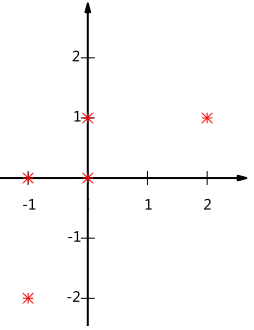现在问题来了：如果我们必须使用一维来表示这些数据，又希望尽量保留原始的信息，你要如何选择？
通过上一节对基变换的讨论我们知道，这个问题实际上是要在二维平面中选择一个方向，将所有数据都投影到这个方向所在直线上，用投影值表示原始记录。这是一个实际的二维降到一维的问题。那么如何选择这个方向（或者说基）才能尽量保留最多的原始信息呢？一种直观的看法是：希望投影后的投影值尽可能分散。以上图为例，可以看出如果向x轴投影，那么最左边的两个点会重叠在一起，中间的两个点也会重叠在一起，于是本身四个各不相同的二维点投影后只剩下两个不同的值了，这是一种严重的信息丢失，同理，如果向y轴投影最上面的两个点和分布在x轴上的两个点也会重叠。所以看来x和y轴都不是最好的投影选择。我们直观目测，如果向通过第一象限和第三象限的斜线投影，则五个点在投影后还是可以区分的。接下来，我们用数学方法表述这个问题。
方差
上文说到，我们希望投影后投影值尽可能分散，而这种分散程度，可以用数学上的方差来表述。此处，一个字段的方差可以看做是每个元素与字段均值的差的平方和的均值，即：

Var(a)=1m∑i=1m(ai−μ)2

由于上面我们已经将每个字段的均值都化为0了，因此方差可以直接用每个元素的平方和除以元素个数表示：

Var(a)=1m∑i=1ma2i

于是上面的问题被形式化表述为：寻找一个一维基，使得所有数据变换为这个基上的坐标表示后，方差值最大。
协方差
对于上面二维降成一维的问题来说，找到那个使得方差最大的方向就可以了。不过对于更高维，还有一个问题需要解决。考虑三维降到二维问题。与之前相同，首先我们希望找到一个方向使得投影后方差最大，这样就完成了第一个方向的选择，继而我们选择第二个投影方向。如果我们还是单纯只选择方差最大的方向，很明显，这个方向与第一个方向应该是“几乎重合在一起”，显然这样的维度是没有用的，因此，应该有其他约束条件。从直观上说，让两个字段尽可能表示更多的原始信息，我们是不希望它们之间存在（线性）相关性的，因为相关性意味着两个字段不是完全独立，必然存在重复表示的信息。
数学上可以用两个字段的协方差表示其相关性，由于已经让每个字段均值为0，则：

Cov(a,b)=1m∑i=1maibi

可以看到，在字段均值为0的情况下，两个字段的协方差简洁的表示为其内积除以元素数m。当协方差为0时，表示两个字段完全独立。为了让协方差为0，我们选择第二个基时只能在与第一个基正交的方向上选择。因此最终选择的两个方向一定是正交的。至此，我们得到了降维问题的优化目标：将一组N维向量降为K维（K大于0，小于N），其目标是选择K个单位（模为1）正交基，使得原始数据变换到这组基上后，各字段两两间协方差为0，而字段的方差则尽可能大（在正交的约束下，取最大的K个方差）。
协方差矩阵
上面我们导出了优化目标，但是这个目标似乎不能直接作为操作指南（或者说算法），因为它只说要什么，但根本没有说怎么做。所以我们要继续在数学上研究计算方案。我们看到，最终要达到的目的与字段内方差及字段间协方差有密切关系。因此我们希望能将两者统一表示，仔细观察发现，两者均可以表示为内积的形式，而内积又与矩阵相乘密切相关。于是我们来了灵感：假设我们只有a和b两个字段，那么我们将它们按行组成矩阵X：

X=(a1b1a2b2⋯⋯ambm)

然后我们用X乘以X的转置，并乘上系数1/m：

1mXXT=⎛⎝⎜⎜⎜⎜⎜1m∑i=1ma2i1m∑i=1maibi1m∑i=1maibi1m∑i=1mb2i⎞⎠⎟⎟⎟⎟⎟

奇迹出现了！这个矩阵对角线上的两个元素分别是两个字段的方差，而其它元素是a和b的协方差。两者被统一到了一个矩阵的。
根据矩阵相乘的运算法则，这个结论很容易被推广到一般情况：设我们有m个n维数据记录，将其按列排成n乘m的矩阵X，设

C=1mXXT
，则C是一个对称矩阵，其对角线分别个各个字段的方差，而第i行j列和j行i列元素相同，表示i和j两个字段的协方差。
协方差矩阵对角化
根据上述推导，我们发现要达到优化目前，等价于将协方差矩阵对角化：即除对角线外的其它元素化为0，并且在对角线上将元素按大小从上到下排列，这样我们就达到了优化目的。这样说可能还不是很明晰，我们进一步看下原矩阵与基变换后矩阵协方差矩阵的关系：设原始数据矩阵X对应的协方差矩阵为C，而P是一组基按行组成的矩阵，设Y=PX，则Y为X对P做基变换后的数据。设Y的协方差矩阵为D，我们推导一下D与C的关系：

D=====1mYYT1m(PX)(PX)T1mPXXTPTP(1mXXT)PTPCPT

现在事情很明白了！我们要找的P不是别的，而是能让原始协方差矩阵对角化的P。换句话说，优化目标变成了寻找一个矩阵P，满足

PCPT
是一个对角矩阵，并且对角元素按从大到小依次排列，那么P的前K行就是要寻找的基，用P的前K行组成的矩阵乘以X就使得X从N维降到了K维并满足上述优化条件。现在所有焦点都聚焦在了协方差矩阵对角化问题上，由上文知道，协方差矩阵C是一个是对称矩阵，在线性代数上，实对称矩阵有一系列非常好的性质：第一、实对称矩阵不同特征值对应的特征向量必然正交；第二、设特征向量

λ
重数为r，则必然存在r个线性无关的特征向量对应于

λ
，因此可以将这r个特征向量单位正交化。由两条性质可知，一个n行n列的实对称矩阵一定可以找到n个单位正交特征向量，设这n个特征向量为

e1,e2,⋯,en
，我们将其按列组成矩阵：

E=(e1e2⋯en)

则对协方差矩阵C有如下结论：

ETCE=Λ=⎛⎝⎜⎜⎜⎜λ1λ2⋱λn⎞⎠⎟⎟⎟⎟

其中

Λ
为对角矩阵，其对角元素为各特征向量对应的特征值(可能有重复)。
因此，我们可以发现已经找到了需要的矩阵P：

P=ET

P是协方差矩阵的特征向量单位化后按行排列出的矩阵，其中每一行都是C的一个特征向量。如果设P按照

Λ
中特征值的从大到小，将特征向量从上到下排列，则用P的前K行组成的矩阵乘以原始数据矩阵X，就得到了我们需要的降维后的数据矩阵Y。

关于Image Engineering & Computer Vision的更多讨论与交流，敬请关注本博客和新浪微博songzi_tea.

展开全文计算机视觉 图像分析
• 协方差矩阵详解以及numpy计算协方差矩阵(np.cov) 协方差矩阵详解 均值，标准差与方差 由简单的统计学基础知识，我们有如下公式： Xˉ=∑i=1nXin\bar X{\rm{ = }}\frac{{\sum\limits_{i = 1}^n {{X_i}} }}{{\rm{n}}}X...
协方差矩阵详解以及numpy计算协方差矩阵(np.cov)
协方差矩阵详解
均值，标准差与方差
由简单的统计学基础知识，我们有如下公式：

X

ˉ

=

∑

i

=

1

n

X

i

n

\bar X{\rm{ = }}\frac{{\sum\limits_{i = 1}^n {{X_i}} }}{{\rm{n}}}

S

=

∑

i

=

1

n

(

X

i

−

X

ˉ

)

2

n

−

1

S = \sqrt {\frac{{\sum\limits_{i = 1}^n {{{({X_i} - \bar X)}^2}} }}{{n - 1}}}

S

2

=

∑

i

=

1

n

(

X

i

−

X

ˉ

)

2

n

−

1

{S^2} = \frac{{\sum\limits_{i = 1}^n {{{({X_i} - \bar X)}^2}} }}{{n - 1}}

其中

X

ˉ

\bar X

是样本均值，反映了n个样本观测值的整体大小情况。

S

S

是样本标准差，反应的是样本的离散程度。标准差越大，数据越分散。

S

2

S^2

是样本方差，是

S

S

的平方。
均值虽然可以在一定程度上反应数据的整体大小，但是仍然不能反应数据的内部离散程度。而标准差和方差弥补了这一点。
但是标准差和方差都是针对一维数组的，即1 x d数组。该数组的行代表的是一个随机变量（可理解为属性），如工资等。每一列代表一个观测值。如果一个事物具有多种属性，即有多个随机变量，那么我们会得到一个var_num x d数组。该数组的每一行都是一个随机变量（属性），每一列代表着一个在这些属性维度上的观测值样本。如果我们想要分析该事物，那么仅仅将其剥离为单独的1 x d去求其标准差是不够的，我们还需要关注这些随机变量（属性）variable内部之间的联系。如工资和年龄的联系，工资和技术水平的联系等。
所以便自然而然的引入了协方差。
协方差
两个随机变量的协方差反映了这两个随机变量一致的分散程度有多大。 通俗的讲，协方差反映了两个随机变量的正负相关关系。 由方差的公式，我们可以类比得出协方差的公式：

v

a

r

(

X

)

=

S

2

=

∑

i

=

1

n

(

X

i

−

X

ˉ

)

(

X

i

−

X

ˉ

)

n

−

1

{\mathop{\rm var}} (X) = {S^2} = \frac{{\sum\limits_{i = 1}^n {({X_i} - \bar X)({X_i} - \bar X)} }}{{n - 1}}

c

o

v

(

X

,

Y

)

=

∑

i

=

1

n

(

X

i

−

X

ˉ

)

(

Y

i

−

Y

ˉ

)

n

−

1

=

E

(

(

X

−

E

(

X

)

)

(

Y

−

E

(

Y

)

)

)

{\mathop{\rm cov}} (X,Y) = \frac{{\sum\limits_{i = 1}^n {({X_i} - \bar X)({Y_i} - \bar Y)} }}{{n - 1}} = E((X - E(X))(Y - E(Y)))

相关系数

ρ

\rho

与协方差直接有如下关系：

ρ

=

C

o

v

(

X

,

Y

)

σ

X

σ

Y

=

E

(

(

X

−

E

(

X

)

)

(

Y

−

E

(

Y

)

)

)

σ

X

σ

Y

=

E

(

(

X

−

E

(

X

)

σ

X

)

(

Y

−

E

(

Y

)

σ

Y

)

\rho = \frac{{Cov(X,Y)}}{{{\sigma _X}{\sigma _Y}}} = \frac{{E((X - E(X))(Y - E(Y)))}}{{{\sigma _X}{\sigma _Y}}} = E((\frac{{X - E(X)}}{{{\sigma _X}}})(\frac{{Y - E(Y)}}{{{\sigma _Y}}})

从上述公式可见，相关系数

ρ

\rho

实际上也是一种特殊的协方差。相关系数是数据X和Y做了归一化

x

=

(

X

−

X

ˉ

)

σ

X

x = \frac{{(X - \bar X)}}{{{\sigma _X}}}

,

y

=

(

Y

−

Y

ˉ

)

σ

Y

y = \frac{{(Y - \bar Y)}}{{{\sigma _Y}}}

之后的协方差。

x

,

y

x,y

的方差为1，期望为0。有：

ρ

(

X

,

Y

)

=

c

o

v

(

x

,

y

)

\rho(X,Y) = cov(x,y)

协方差的意义此时应该很清晰了。
协方差矩阵
对于具有很多个随机变量的数据，随机变量之间两两都具有一个协方差，这样便形成了一个协方差矩阵。 假设我们有一组数据，其具有三个随机变量，n个观测值：那么其协方差矩阵为：我们可以使用一种便捷的矩阵乘法来计算协方差矩阵。设原数据数组为

X

X

。先对X进行处理，求X每一个随机变量的均值。然后对于每一行，减去该行随机变量的均值，得到

X

′

X^{'}

，记协方差矩阵为

M

M

，那么就有:

M

=

X

′

X

′

T

n

−

1

M = \frac{{X^{'}{X^{'}}^{T}}}{{n-1}}

用代码描述可能更加清晰:
a = np.array([[1,2,3],[4,5,7]])
cov1 = np.cov(a)
mean_a = np.mean(a,axis=1,keepdims=True)
tmpa = a-mean_a
cov2 = np.matmul(tmpa,tmpa.T)/(tmpa.shape-1)
print(cov1)
print(cov2)numpy计算协方差矩阵np.cov()
语法
numpy.cov(m,y=None,rowvar=True,bias=False,ddof=None,fweights=None,aweights=None,dtype) 用于计算给定矩阵和权值的协方差矩阵。
Parameters
m:array_like

A 1-D or 2-D array containing multiple variables and observations. Each row of m represents a variable, and each column a single observation of all those variables. Also see rowvar below.

一维或者二维数组，包含有多个随机变量和观测值。m的每一行代表一个随机变量，每一列代表包含所有随机变量的一个观测值。当给一维数组时，相当于计算的就是方差。
y:array_like,optional

An additional set of variables and observations. y has the same form as that of m.

额外的一组数据，y必须在在数据形式上与m一致。 如果m.shape = (var_num, obs_num)，那么y.shape必须在第二维观测值个数上，即shape与m保持一致，即y也得有obs_num个观测值。实际执行时，会先将这两组数据concatenate，然后再求解。
Example
a = np.array([[1,2,3],[4,5,7]])
b = np.array([[1,2,3,4],[4,5,6,7]])
cov = np.cov(a,b)

执行结果：从执行结果上可见，报错。报错的具体描述便是，两组数据在dimension1不一致。 我们也可以从numpy.cov()源码中看到：
if y is not None:
y = array(y, copy=False, ndmin=2, dtype=dtype)
if not rowvar and y.shape != 1:
y = y.T
X = np.concatenate((X, y), axis=0)

可见是对其进行了concatenate.
bias: bool, optional

Default normalization (False) is by (N - 1), where N is the number of observations given (unbiased estimate). If bias is True, then normalization is by N. These values can be overridden by using the keyword ddof in numpy versions >= 1.5

默认的采用无偏估计，即除以(N-1)，N是样本个数。可以被ddof所覆盖。
rowvar : bool, optional

If rowvar is True (default), then each row represents a variable, with observations in the columns. Otherwise, the relationship is transposed: each column represents a variable, while the rows contain observations.

rowvar指定了行列谁为随机变量的问题。默认为True，即行代表一个随机变量。而列代表观测值。如果为False，那么列代表随机变量，而行代表观测值。
ddof : int, optional

If not None the default value implied by bias is overridden. Note that ddof=1 will return the unbiased estimate, even if both fweights and aweights are specified, and ddof=0 will return the simple average. See the notes for the details. The default value is None.

.. versionadded:: 1.5

ddof，duplicated degrees of freedom，即重复无效的自由度。参见源码详解。
fweights : array_like, int, optional

1-D array of integer frequency weights; the number of times each observation vector should be repeated.

.. versionadded:: 1.10

一维int数组，shape应当与数据的观测值个数一致(即当rowvar=True时候的shape)。指定每个观测值的频率权重，即这个观测值向量(column)应该被重复计算几次。
aweights : array_like, optional

1-D array of observation vector weights. These relative weights are typically large for observations considered “important” and smaller for observations considered less “important”. If ddof=0 the array of weights can be used to assign probabilities to observation vectors.

.. versionadded:: 1.10

一维数组，其shape同样的，应该与观测值个数一致。指定的是每个计算权重，即较重要的观测值其aweight大一些，不那么重要的可以小一些。当ddof为0的时候，相当于观测值的概率。
Return：
out: ndarray: The covariance matrix of the variables.
Example
由于不太直观，所以不举例。分析一下源码。
源码
    if ddof is not None and ddof != int(ddof):   # 这里说明ddof必须是int类型
raise ValueError(
"ddof must be integer")

# Handles complex arrays too
m = np.asarray(m)       # 所以m的输入类型可以是lists, lists of tuples
#tuples, tuples of tuples, tuples of lists and ndarrays.
if m.ndim > 2:          # 不能超过两维
raise ValueError("m has more than 2 dimensions")

if y is None:           # 如果y是None，返回数组类型取原数组类型
# 与np.float64精度高的那一个。
dtype = np.result_type(m, np.float64)
else:                   # 有y输入则先处理y，判断y的维度，再判断数据类型
y = np.asarray(y)
if y.ndim > 2:
raise ValueError("y has more than 2 dimensions")
dtype = np.result_type(m, y, np.float64)

X = array(m, ndmin=2, dtype=dtype)
if not rowvar and X.shape != 1:  # 如果rowvar为False就转置
X = X.T
if X.shape == 0:
return np.array([]).reshape(0, 0)
if y is not None:                    # 对y进行处理
y = array(y, copy=False, ndmin=2, dtype=dtype)
if not rowvar and y.shape != 1:  # 判断rowvar是否转置
y = y.T
X = np.concatenate((X, y), axis=0)  # concatenate

if ddof is None:            # 如果未指定ddof
if bias == 0:           # 如果指定了bias=0,ddof=1,无偏
ddof = 1
else:                   # 否则ddof=0
ddof = 0

# Get the product of frequencies and weights
w = None
if fweights is not None:
fweights = np.asarray(fweights, dtype=float)
if not np.all(fweights == np.around(fweights)):  # round进行取整
# 取整后判断是否全部相等，来判断全都是整数，必须全是整数，否则报错
raise TypeError(
"fweights must be integer")
if fweights.ndim > 1:  # 必须一维
raise RuntimeError(
"cannot handle multidimensional fweights")
if fweights.shape != X.shape: # 必须与观测数一致
raise RuntimeError(
"incompatible numbers of samples and fweights")
if any(fweights < 0):   #必须全部为正值
raise ValueError(
"fweights cannot be negative")
w = fweights        # 将fweight赋给w
if aweights is not None:
aweights = np.asarray(aweights, dtype=float)
if aweights.ndim > 1:
raise RuntimeError(
"cannot handle multidimensional aweights")
if aweights.shape != X.shape:
raise RuntimeError(
"incompatible numbers of samples and aweights")
if any(aweights < 0):
raise ValueError(
"aweights cannot be negative")
if w is None:
w = aweights    # 如果fweight为空，就直接把aweight赋给w
else:
w *= aweights   # 否则w = fweight * aweight

avg, w_sum = average(X, axis=1, weights=w, returned=True)
# 以列为操作单元，求每一个随便变量的所有观测值在权重w下的均值。
# w_sum为w的所有元素的和（权重和）。
w_sum = w_sum

# Determine the normalization
if w is None:       # 如果w为None，那么直接用X的观测值个数（列数）减ddof
fact = X.shape - ddof
elif ddof == 0:  # w不为空，ddof等于0，需要除以的分母就是 w_sum
fact = w_sum
elif aweights is None: # w不为空，aweight为空，ddof不为0
# 直接用 w_sum-ddof(因为此时的w_sum就相当于重复后的观测值个数)
fact = w_sum - ddof
else:   # w不为空，aweight也不为空， fweight也不为空，ddof != 0
# fact就相当于w_sum减去以w为权重的aweight的平均值乘以ddof
# 当aweigth=None的时候，是这个公式的一个特殊情况
# 在这里猜测：ddof： duplicated degreeds of freedom
# 即重复无效的自由度
fact = w_sum - ddof*sum(w*aweights)/w_sum

if fact <= 0:
warnings.warn("Degrees of freedom <= 0 for slice",
RuntimeWarning, stacklevel=3)
fact = 0.0

X -= avg[:, None]   # X减去均值
if w is None:
X_T = X.T
else:
X_T = (X*w).T   # 乘以权重
c = dot(X, X_T.conj())  # X 乘以 X的转置的复共轭矩阵（对标量而言就是转置）
c *= np.true_divide(1, fact)    # 再除以fact
return c.squeeze()  # 删去c中dim为1的维度，输出。

以上就是我对np.cov()的全部解读。

如果觉得文章对您有帮助，可以点个赞，是对作者最大的鼓励。
展开全文python numpy
• 图像协方差矩阵by hadrienj 由hadrienj The goal of this post is to go from the basics of data preprocessing to modern techniques used in deep learning. My point is that we can use code (such as Python/...

图像协方差矩阵
The goal of this post is to go from the basics of data preprocessing to modern techniques used in deep learning. My point is that we can use code (such as Python/NumPy) to better understand abstract mathematical notions. Thinking by coding! ?
这篇文章的目标是从数据预处理的基础知识到深度学习中使用的现代技术。 我的观点是，我们可以使用代码(例如Python / NumPy)更好地理解抽象的数学概念。 通过编码思考！ ？
We will start with basic but very useful concepts in data science and machine learning/deep learning, like variance and covariance matrices. We will go further to some preprocessing techniques used to feed images into neural networks. We will try to get more concrete insights using code to actually see what each equation is doing.
我们将从数据科学和机器学习/深度学习中的基本但非常有用的概念入手，例如方差和协方差矩阵。 我们将进一步介绍一些用于将图像馈入神经网络的预处理技术。 我们将尝试使用代码来获得更具体的见解，以实际查看每个方程式在做什么。
Preprocessing refers to all the transformations on the raw data before it is fed to the machine learning or deep learning algorithm. For instance, training a convolutional neural network on raw images will probably lead to bad classification performances (Pal & Sudeep, 2016). The preprocessing is also important to speed up training (for instance, centering and scaling techniques, see Lecun et al., 2012; see 4.3).
预处理是指对原始数据进行的所有转换，然后再将其转换为机器学习或深度学习算法。 例如，在原始图像上训练卷积神经网络可能会导致分类性能不佳( Pal＆Sudeep，2016 )。 预处理对于加快训练速度也很重要(例如，定心和缩放技术，请参阅Lecun等人，2012；请参阅4.3 )。
Here is the syllabus of this tutorial:
这是本教程的课程表：
1. Background: In the first part, we will get some reminders about variance and covariance. We will see how to generate and plot fake data to get a better understanding of these concepts.
1.背景：在第一部分中，我们将提醒您一些方差和协方差。 我们将看到如何生成和绘制虚假数据以更好地理解这些概念。
2. Preprocessing: In the second part we will see the basics of some preprocessing techniques that can be applied to any kind of data — mean normalization, standardization, and whitening.
2.预处理：在第二部分中，我们将看到可应用于任何类型数据的一些预处理技术的基础- 均值归一化 ， 标准化和白化 。
3. Whitening images: In the third part, we will use the tools and concepts gained in 1. and 2. to do a special kind of whitening called Zero Component Analysis (ZCA). It can be used to preprocess images for deep learning. This part will be very practical and fun ☃️!
3.增白图像：在第三部分中，我们将使用在1.和2.中获得的工具和概念来进行一种特殊的增白，称为零分量分析 (ZCA)。 它可以用于预处理图像以进行深度学习。 这部分将非常实用且有趣☃️！
Feel free to fork the notebook associated with this post! For instance, check the shapes of the matrices each time you have a doubt.
随意分叉与此帖子相关的笔记本 ！ 例如，每当您有疑问时，请检查矩阵的形状。
1.背景 (1. Background)
A.方差和协方差 (A. Variance and covariance)
The variance of a variable describes how much the values are spread. The covariance is a measure that tells the amount of dependency between two variables.
变量的方差描述了值的分散程度。 协方差是衡量两个变量之间依存度的量度。
A positive covariance means that the values of the first variable are large when values of the second variables are also large. A negative covariance means the opposite: large values from one variable are associated with small values of the other.
正协方差意味着当第二变量的值也很大时，第一变量的值就很大。 负协方差则相反：一个变量的大值与另一个变量的小值相关。
The covariance value depends on the scale of the variable so it is hard to analyze it. It is possible to use the correlation coefficient that is easier to interpret. The correlation coefficient is just the normalized covariance.
协方差值取决于变量的大小，因此很难对其进行分析。 可以使用更容易解释的相关系数。 相关系数只是归一化协方差。
The covariance matrix is a matrix that summarises the variances and covariances of a set of vectors and it can tell a lot of things about your variables. The diagonal corresponds to the variance of each vector:
协方差矩阵是一个汇总一组向量的方差和协方差的矩阵，它可以告诉您很多有关变量的信息。 对角线对应于每个向量的方差：
Let’s just check with the formula of the variance:
让我们检查方差的公式：
with n the length of the vector, and x̄ the mean of the vector. For instance, the variance of the first column vector of A is:
其中向量的长度为n ，向量的平均值为x̄ 。 例如， A的第一列向量的方差为：
This is the first cell of our covariance matrix. The second element on the diagonal corresponds of the variance of the second column vector from A and so on.
这是我们协方差矩阵的第一个单元格。 对角线上的第二个元素对应于第二列向量相对于A的方差，依此类推。
Note: the vectors extracted from the matrix A correspond to the columns of A.
注意 ：从所述矩阵A对应于A的列中提取的矢量。
The other cells correspond to the covariance between two column vectors from A. For instance, the covariance between the first and the third column is located in the covariance matrix as the column 1 and the row 3 (or the column 3 and the row 1).
其他像元对应于来自A的两个列向量之间的协方差。 例如，第一列和第三列之间的协方差位于协方差矩阵中，作为列1和行3(或列3和行1)。
Let’s check that the covariance between the first and the third column vector of A is equal to -2.67. The formula of the covariance between two variables X and Y is:
我们检查A的第一列向量和第三列向量之间的协方差是否等于-2.67。 两个变量X和Y之间的协方差公式为：
The variables X and Y are the first and the third column vectors in the last example. Let’s split this formula to be sure that it is crystal clear:
变量X和Y是最后一个示例中的第一列向量和第三列向量。 让我们对这个公式进行拆分，以确保它非常清晰：
The sum symbol (Σ) means that we will iterate on the elements of the vectors. We will start with the first element (i=1) and calculate the first element of X minus the mean of the vector X. 和符号( Σ )表示我们将迭代向量的元素。 我们将从第一个元素( i = 1 )开始，然后计算X的第一个元素减去向量X的平均值。
2. Multiply the result with the first element of Y minus the mean of the vector Y.
2.将结果乘以Y的第一个元素减去向量Y的平均值。
3. Reiterate the process for each element of the vectors and calculate the sum of all results.
3.对向量的每个元素重复该过程，并计算所有结果的总和。
4. Divide by the number of elements in the vector.
4.除以向量中元素的数量。
Example 1.
范例1。
让我们从矩阵A开始：
We will calculate the covariance between the first and the third column vectors:
我们将计算第一列向量和第三列向量之间的协方差：
and
和
x̄=3, ȳ=4, and n=3 so we have:
x̄ = 3 ， ȳ= 4和n = 3，所以我们有：
Ok, great! That’s the value of the covariance matrix.
好的太棒了！ 那就是协方差矩阵的值。
Now the easy way. With NumPy, the covariance matrix can be calculated with the function np.cov.
现在简单的方法 。 使用NumPy，可以使用函数np.cov计算协方差矩阵。
It is worth noting that if you want NumPy to use the columns as vectors, the parameter rowvar=False has to be used. Also, bias=True divides by n and not by n-1.
值得注意的是 ，如果希望NumPy将列用作向量，则必须使用参数rowvar=False 。 此外， bias=True除以n   而不是n-1 。
Let’s create the array first:
让我们首先创建数组：
array([[1, 3, 5],       [5, 4, 1],       [3, 8, 6]])
Now we will calculate the covariance with the NumPy function:
现在，我们将使用NumPy函数计算协方差：
array([[ 2.66666667, 0.66666667, -2.66666667],       [ 0.66666667, 4.66666667, 2.33333333],       [-2.66666667, 2.33333333, 4.66666667]])
Looks good!
看起来挺好的！
Finding the covariance matrix with the dot product
用点积找到协方差矩阵
There is another way to compute the covariance matrix of A. You can center A around 0. The mean of the vector is subtracted from each element of the vector to have a vector with mean equal to 0. It is multiplied with its own transpose, and divided by the number of observations.
还有另一种计算A的协方差矩阵的方法。 您可以将A围绕0居中。从向量的每个元素中减去向量的平均值，得到一个向量的平均值等于0。将其乘以自己的转置，然后除以观察次数。
Let’s start with an implementation and then we’ll try to understand the link with the previous equation:
让我们从一个实现开始，然后我们将尝试理解与前面的等式的链接：
Let’s test it on our matrix A:
让我们在矩阵A上进行测试：
array([[ 2.66666667, 0.66666667, -2.66666667],       [ 0.66666667, 4.66666667, 2.33333333],       [-2.66666667, 2.33333333, 4.66666667]])
We end up with the same result as before.
我们最终得到与以前相同的结果。
The explanation is simple. The dot product between two vectors can be expressed:
解释很简单。 两个向量之间的点积可以表示为：
That’s right, it is the sum of the products of each element of the vectors:
没错，它是向量每个元素的乘积之和：
If n is the number of elements in our vectors and that we divide by n:
如果n是向量中元素的数量，并且我们除以n ：
You can note that this is not too far from the formula of the covariance we have seen earlier:
您可以注意到，这与我们之前看到的协方差公式相差不大：
The only difference is that, in the covariance formula, we subtract the mean of a vector from each of its elements. This is why we need to center the data before doing the dot product.
唯一的区别是，在协方差公式中，我们从向量的每个元素中减去向量的平均值。 这就是为什么我们需要在进行点积运算之前将数据居中。
Now, if we have a matrix A, the dot product between A and its transpose will give you a new matrix:
现在，如果我们有一个矩阵A ，则A及其转置之间的点积将为您提供一个新的矩阵：
This is the covariance matrix!
这是协方差矩阵！
B.可视化数据和协方差矩阵 (B. Visualize data and covariance matrices)
In order to get more insights about the covariance matrix and how it can be useful, we will create a function to visualize it along with 2D data. You will be able to see the link between the covariance matrix and the data.
为了获得有关协方差矩阵及其有用性的更多见解，我们将创建一个函数以将其与2D数据一起可视化。 您将能够看到协方差矩阵与数据之间的链接。
This function will calculate the covariance matrix as we have seen above. It will create two subplots — one for the covariance matrix and one for the data. The heatmap() function from Seaborn is used to create gradients of colour — small values will be coloured in light green and large values in dark blue. We chose one of our palette colours, but you may prefer other colours. The data is represented as a scatterplot.
如上所述，该函数将计算协方差矩阵。 它将创建两个子图-一个用于协方差矩阵，另一个用于数据。 Seaborn的heatmap()函数用于创建颜色渐变-小值将用浅绿色着色，大值将用深蓝色着色。 我们选择了一种调色板颜色，但您可能更喜欢其他颜色。 数据表示为散点图。
C.模拟数据 (C. Simulating data)
Uncorrelated data
不相关的数据
Now that we have the plot function, we will generate some random data to visualize what the covariance matrix can tell us. We will start with some data drawn from a normal distribution with the NumPy function np.random.normal().
现在有了图函数，我们将生成一些随机数据以可视化协方差矩阵可以告诉我们的内容。 我们将从使用NumPy函数np.random.normal()从正态分布中提取的一些数据开始。
This function needs the mean, the standard deviation and the number of observations of the distribution as input. We will create two random variables of 300 observations with a standard deviation of 1. The first will have a mean of 1 and the second a mean of 2. If we randomly draw two sets of 300 observations from a normal distribution, both vectors will be uncorrelated.
该函数需要均值，标准差和分布的观察次数作为输入。 我们将创建两个300个观察值的随机变量，标准偏差为1。第一个变量的平均值为1，第二个变量的平均值为2。如果我们从正态分布中随机抽取两组300个观察值，则两个向量均为不相关的。
(300, 2)
Note 1: We transpose the data with .T because the original shape is (2, 300) and we want the number of observations as rows (so with shape (300, 2)).
注1 ：因为原始形状为(2, 300) .T (2, 300)并且我们希望观察的数目为行(因此形状为(300, 2) ) (300, 2)所以我们使用.T转置数据。
Note 2: We use np.random.seed function for reproducibility. The same random number will be used the next time we run the cell.
注意2 ：我们使用np.random.seed函数来提高可重复性。 下次运行单元时，将使用相同的随机数。
Let’s check how the data looks like:
让我们检查数据的外观：
array([[ 2.47143516, 1.52704645],       [ 0.80902431, 1.7111124 ],       [ 3.43270697, 0.78245452],       [ 1.6873481 , 3.63779121],       [ 1.27941127, -0.74213763],       [ 2.88716294, 0.90556519],       [ 2.85958841, 2.43118375],       [ 1.3634765 , 1.59275845],       [ 2.01569637, 1.1702969 ],       [-0.24268495, -0.75170595]])
Nice, we have two column vectors.
好的，我们有两个列向量。
Now, we can check that the distributions are normal:
现在，我们可以检查分布是否正常：
Looks good!
看起来挺好的！
We can see that the distributions have equivalent standard deviations but different means (1 and 2). So that’s exactly what we have asked for.
我们可以看到分布具有相等的标准偏差，但均值不同(1和2)。 这正是我们所要求的。
Now we can plot our dataset and its covariance matrix with our function:
现在，我们可以使用函数绘制数据集及其协方差矩阵：
Covariance matrix:[[ 0.95171641 -0.0447816 ] [-0.0447816 0.87959853]]
We can see on the scatterplot that the two dimensions are uncorrelated. Note that we have one dimension with a mean of 1 (y-axis) and the other with the mean of 2 (x-axis).
我们可以在散点图上看到这两个维度是不相关的。 请注意，我们有一维的平均值为1(y轴)，另一维的平均值为2(x轴)。
Also, the covariance matrix shows that the variance of each variable is very large (around 1) and the covariance of columns 1 and 2 is very small (around 0). Since we ensured that the two vectors are independent this is coherent. The opposite is not necessarily true: a covariance of 0 doesn’t guarantee independence (see here).
同样，协方差矩阵显示每个变量的方差非常大(大约1)，列1和2的协方差很小(大约0)。 因为我们确保两个向量是独立的，所以这是相干的。 相反的情况不一定成立：协方差0不能保证独立性(请参见此处 )。
Correlated data
相关数据
Now, let’s construct dependent data by specifying one column from the other one.
现在，让我们通过指定另一列中的一列来构造依赖数据。
Covariance matrix:[[ 0.95171641 0.92932561] [ 0.92932561 1.12683445]]
The correlation between the two dimensions is visible on the scatter plot. We can see that a line could be drawn and used to predict y from x and vice versa. The covariance matrix is not diagonal (there are non-zero cells outside of the diagonal). That means that the covariance between dimensions is non-zero.
二维关系在散点图上可见。 我们可以看到一条线可以用来从x预测y ，反之亦然。 协方差矩阵不是对角线(对角线之外有非零像元)。 这意味着维度之间的协方差不为零。
That’s great! We now have all the tools to see different preprocessing techniques.
那很棒！ 现在，我们拥有所有工具来查看不同的预处理技术。
2.预处理 (2. Preprocessing)
A.平均归一化 (A. Mean normalization)
Mean normalization is just removing the mean from each observation.
均值归一化只是从每个观察值中除去均值。
where X’ is the normalized dataset, X is the original dataset, and x̅ is the mean of X.
其中X'是归一化数据集， X是原始数据集， x̅是X的均值。
Mean normalization has the effect of centering the data around 0. We will create the function center() to do that:
均值归一化的作用是将数据以0为中心。我们将创建函数center()来做到这一点：
Let’s give it a try with the matrix B we have created earlier:
让我们尝试一下我们之前创建的矩阵B ：
Before:
Covariance matrix:[[ 0.95171641 0.92932561] [ 0.92932561 1.12683445]]
After:
Covariance matrix:[[ 0.95171641 0.92932561] [ 0.92932561 1.12683445]]
The first plot shows again the original data B and the second plot shows the centered data (look at the scale).
第一个图再次显示了原始数据B ，第二个图显示了居中的数据(看比例尺)。
B.标准化或规范化 (B. Standardization or normalization)
Standardization is used to put all features on the same scale. Each zero-centered dimension is divided by its standard deviation.
标准化用于将所有功能置于相同的比例。 每个零中心尺寸均除以其标准偏差。
where X’ is the standardized dataset, X is the original dataset, x̅ is the mean of X, and σ is the standard deviation of X.
其中X'是标准化数据集， X是原始数据集， x̅是X的平均值，   σ是X的标准偏差。
Let’s create another dataset with a different scale to check that it is working.
让我们创建另一个具有不同比例的数据集以检查其是否正常工作。
Covariance matrix:[[ 0.95171641 0.83976242] [ 0.83976242 6.22529922]]
We can see that the scales of x and y are different. Note also that the correlation seems smaller because of the scale differences. Now let’s standardize it:
我们可以看到x和y的比例不同。 还要注意，由于比例差异，相关性似乎较小。 现在让我们对其进行标准化：
Covariance matrix:[[ 1.          0.34500274] [ 0.34500274  1.        ]]
Looks good. You can see that the scales are the same and that the dataset is zero-centered according to both axes.
看起来挺好的。 您可以看到比例是相同的，并且根据两个轴，数据集都是零中心的。
Now, have a look at the covariance matrix. You can see that the variance of each coordinate — the top-left cell and the bottom-right cell — is equal to 1.
现在，看一下协方差矩阵。 您可以看到每个坐标(左上角的单元格和右下角的单元格)的方差等于1。
This new covariance matrix is actually the correlation matrix. The Pearson correlation coefficient between the two variables (c1 and c2) is 0.54220151.
这个新的协方差矩阵实际上是相关矩阵。 两个变量( c1和c2 )之间的皮尔逊相关系数为0.54220151。
C.美白 (C. Whitening)
Whitening, or sphering, data means that we want to transform it to have a covariance matrix that is the identity matrix — 1 in the diagonal and 0 for the other cells. It is called whitening in reference to white noise.
数据变白或变圆意味着我们要对其进行转换以使其具有协方差矩阵，该矩阵为恒等矩阵-对角线为1，其他单元格为0。 关于白噪声，这称为白化。
Here are more details on the identity matrix.
这是有关身份矩阵的更多详细信息。
Whitening is a bit more complicated than the other preprocessing, but we now have all the tools that we need to do it. It involves the following steps:
增白比其他预处理要复杂一些，但是现在我们拥有完成此过程所需的所有工具。 它涉及以下步骤：
Zero-center the data 零中心数据 Decorrelate the data 解相关数据 Rescale the data 重新缩放数据
Let’s take again C and try to do these steps.
让我们再次使用C并尝试执行这些步骤。
Zero-centering 零中心
This refers to mean normalization (2. A). Check back for details about the center() function.
这是指平均归一化( 2. A )。 请返回以获取有关center()函数的详细信息。
Covariance matrix:[[ 0.95171641  0.83976242] [ 0.83976242  6.22529922]]
2. Decorrelate
2.去相关
At this point, we need to decorrelate our data. Intuitively, it means that we want to rotate the data until there is no correlation anymore. Look at the following image to see what I mean:
此时，我们需要对数据进行解相关。 直观地讲，这意味着我们要旋转数据，直到不再相关为止。 查看下图以了解我的意思：
The left plot shows correlated data. For instance, if you take a data point with a big x value, chances are that the associated y will also be quite big.
左图显示了相关数据。 例如，如果采用x值较大的数据点，则关联的y可能也会很大。
Now take all data points and do a rotation (maybe around 45 degrees counterclockwise. The new data, plotted on the right, is not correlated anymore. You can see that big and small y values are related to the same kind of x values.
现在获取所有数据点并进行旋转(可能逆时针大约45度。在右侧绘制的新数据不再相关。您可以看到，大和小的y值与同一种x值相关。
The question is: how could we find the right rotation in order to get the uncorrelated data?
问题是：我们如何才能找到正确的轮换以获得不相关的数据？
Actually, it is exactly what the eigenvectors of the covariance matrix do. They indicate the direction where the spread of the data is at its maximum:
实际上，这正是协方差矩阵的特征向量所做的。 它们指示最大数据传播方向：
The eigenvectors of the covariance matrix give you the direction that maximizes the variance. The direction of the green line is where the variance is maximum. Just look at the smallest and largest point projected on this line — the spread is big. Compare that with the projection on the orange line — the spread is very small.
协方差矩阵的特征向量为您提供了使方差最大化的方向。 绿线的方向是方差最大的地方。 只要看一下这条线上投影的最小和最大点，点差就会很大。 将其与橙色线上的投影进行比较-点差很小。
For more details about eigendecomposition, see this post.
有关本征分解的更多详细信息，请参阅这篇文章 。
So we can decorrelate the data by projecting it using the eigenvectors. This will have the effect to apply the rotation needed and remove correlations between the dimensions. Here are the steps:
因此，我们可以使用特征向量对数据进行解相关。 这样可以有效地应用所需的旋转并消除尺寸之间的相关性。 步骤如下：
Calculate the covariance matrix 计算协方差矩阵 Calculate the eigenvectors of the covariance matrix 计算协方差矩阵的特征向量 Apply the matrix of eigenvectors to the data — this will apply the rotation 将特征向量矩阵应用于数据-这将应用旋转  Let’s pack that into a function: 让我们将其打包为一个函数：
Let’s try to decorrelate our zero-centered matrix C to see it in action:
让我们尝试对以零为中心的矩阵C进行解相关以查看其作用：
Covariance matrix:[[ 0.95171641 0.83976242] [ 0.83976242 6.22529922]]
Covariance matrix:[[ 5.96126981e-01 -1.48029737e-16] [ -1.48029737e-16 3.15205774e+00]]
Nice! This is working.
真好！ 可以了
We can see that the correlation is not here anymore. The covariance matrix, now a diagonal matrix, confirms that the covariance between the two dimensions is equal to 0.
我们可以看到相关性不再存在。 协方差矩阵(现为对角矩阵)确认两个维度之间的协方差等于0。
3. Rescale the data
3.重新缩放数据
The next step is to scale the uncorrelated matrix in order to obtain a covariance matrix corresponding to the identity matrix.To do that, we scale our decorrelated data by dividing each dimension by the square-root of its corresponding eigenvalue.
下一步是缩放不相关矩阵，以获得与恒等矩阵相对应的协方差矩阵，为此，我们将每个维度除以其对应特征值的平方根来缩放去相关数据。
Note: we add a small value (here 10^-5) to avoid division by 0.
注意 ：我们添加一个较小的值(此处为10 ^ -5)，以避免被0除。
Covariance matrix:[[ 9.99983225e-01 -1.06581410e-16] [ -1.06581410e-16 9.99996827e-01]]
Hooray! We can see that with the covariance matrix that this is all good. We have something that looks like an identity matrix — 1 on the diagonal and 0 elsewhere.
万岁！ 我们可以看到，通过协方差矩阵，这一切都很好。 我们有一个看起来像恒等矩阵的东西-对角线上为1，其他地方为0。
3.图像变白 (3. Image whitening)
We will see how whitening can be applied to preprocess an image dataset. To do so we will use the paper of Pal & Sudeep (2016) where they give some details about the process. This preprocessing technique is called Zero component analysis (ZCA).
我们将看到如何将增白应用于图像数据集的预处理。 为此，我们将使用Pal＆Sudeep(2016)的论文，其中提供有关该过程的一些详细信息。 这种预处理技术称为零成分分析(ZCA)。
Check out the paper, but here is the kind of result they got. The original images (left) and the images after the ZCA (right) are shown.
查看本文，但这是他们得到的结果。 显示了原始图像(左)和ZCA之后的图像(右)。
First things first. We will load images from the CIFAR dataset. This dataset is available from Keras and you can also download it here.
首先是第一件事。 我们将从CIFAR数据集中加载图像。 该数据集可从Keras获得，也可以在此处下载。
(50000, 32, 32, 3)
The training set of the CIFAR10 dataset contains 50000 images. The shape of X_train is (50000, 32, 32, 3). Each image is 32px by 32px and each pixel contains 3 dimensions (R, G, B). Each value is the brightness of the corresponding color between 0 and 255.
CIFAR10数据集的训练集包含50000张图像。 X_train的形状是(50000, 32, 32, 3) X_train (50000, 32, 32, 3) 。 每个图像为32px x 32px，每个像素包含3个尺寸(R，G，B)。 每个值是0到255之间的相应颜色的亮度。
We will start by selecting only a subset of the images, let’s say 1000:
我们将从仅选择图像的子集开始，比如说1000：
(1000, 32, 32, 3)
That’s better. Now we will reshape the array to have flat image data with one image per row. Each image will be (1, 3072) because 32 x 32 x 3 = 3072. Thus, the array containing all images will be (1000, 3072):
这样更好 现在，我们将对数组进行整形，使其具有平面图像数据，每行一幅图像。 每个图像将是(1, 3072)因为32 x 32 x 3 =3072。因此，包含所有图像的数组将是(1000, 3072) ：
(1000, 3072)
The next step is to be able to see the images. The function imshow() from Matplotlib (doc) can be used to show images. It needs images with the shape (M x N x 3) so let’s create a function to reshape the images and be able to visualize them from the shape (1, 3072).
下一步是能够看到图像。 Matplotlib( doc )中的函数imshow()可用于显示图像。 它需要形状为(M x N x 3)的图像，因此让我们创建一个函数来重塑图像并能够从形状(1, 3072)可视化它们。
For instance, let’s plot one of the images we have loaded:
例如，让我们绘制我们已加载的图像之一：
Cute!
可爱！
We can now implement the whitening of the images. Pal & Sudeep (2016) describe the process:
现在，我们可以实现图像的白化。 Pal＆Sudeep(2016)描述了这一过程：
1. The first step is to rescale the images to obtain the range [0, 1] by dividing by 255 (the maximum value of the pixels).
1.第一步是通过除以255(像素的最大值)来重新缩放图像以获得范围[0，1]。
Recall that the formula to obtain the range [0, 1] is:
回想一下获得范围[0，1]的公式为：
but, here, the minimum value is 0, so this leads to:
但是这里的最小值是0，因此导致：
X.min() 0.0X.max() 1.0
Mean subtraction: per-pixel or per-image?
平均减法：按像素还是按图像？
Ok cool, the range of our pixel values is between 0 and 1 now. The next step is:
好的，我们的像素值范围现在在0和1之间。 下一步是：
2. Subtract the mean from all images.
2.从所有图像中减去均值。
Be careful here.
小心点
One way to do it is to take each image and remove the mean of this image from every pixel (Jarrett et al., 2009). The intuition behind this process is that it centers the pixels of each image around 0.
一种方法是拍摄每个图像，然后从每个像素中去除该图像的均值( Jarrett等，2009 )。 此过程的直觉是将每个图像的像素居中于0左右。
Another way to do it is to take each of the 3072 pixels that we have (32 by 32 pixels for R, G and B) for every image and subtract the mean of that pixel across all images. This is called per-pixel mean subtraction. This time, each pixel will be centered around 0 according to all images. When you will feed your network with the images, each pixel is considered as a different feature. With the per-pixel mean subtraction, we have centered each feature (pixel) around 0. This technique is commonly used (e.g Wan et al., 2013).
另一种方法是获取每个图像的每个像素3072个像素(R，G和B分别为32 x 32像素)，然后减去所有图像中该像素的平均值。 这称为每像素均值减法。 这次， 根据所有图像 ，每个像素将以0为中心。 当您向网络提供图像时，每个像素被视为一个不同的功能。 通过逐像素均值减法，我们将每个特征(像素)的中心定在0左右。此技术是常用的(例如Wan等人，2013年 )。
We will now do the per-pixel mean subtraction from our 1000 images. Our data are organized with these dimensions (images, pixels). It was (1000, 3072) because there are 1000 images with 32 x 32 x 3 = 3072 pixels. The mean per-pixel can thus be obtained from the first axis:
现在，我们将从1000张图像中进行每像素均值减法。 我们的数据按照这些维度(images, pixels) 。 之所以是(1000, 3072) ，是因为有1000张图像的32 x 32 x 3 = 3072像素。 因此，可以从第一个轴获得每个像素的平均值：
(3072,)
This gives us 3072 values which is the number of means — one per pixel. Let’s see the kind of values we have:
这提供了3072个值，即均值数量-每个像素一个。 让我们看看我们拥有的价值类型：
array([ 0.5234 , 0.54323137, 0.5274 , …, 0.50369804, 0.50011765, 0.45227451])
This is near 0.5 because we already have normalized to the range [0, 1]. However, we still need to remove the mean from each pixel:
这接近0.5，因为我们已经将范围归一化为[0，1]。 但是，我们仍然需要删除每个像素的均值：
Just to convince ourselves that it worked, we will compute the mean of the first pixel. Let’s hope that it is 0.
只是为了让自己确信它起作用，我们将计算第一个像素的均值。 希望它是0。
array([ -5.30575583e-16, -5.98021632e-16, -4.23439062e-16, …, -1.81965554e-16, -2.49800181e-16, 3.98570066e-17])
This is not exactly 0 but it is small enough that we can consider that it worked!
这不完全是0，但足够小，我们可以认为它起作用了！
Now we want to calculate the covariance matrix of the zero-centered data. Like we have seen above, we can calculate it with the np.cov() function from NumPy.
现在我们要计算零中心数据的协方差矩阵。 就像我们在上面看到的，我们可以使用NumPy中的np.cov()函数进行计算。
Please note that our variables are our different images. This implies that the variables are the rows of the matrix X. Just to be clear, we will tell this information to NumPy with the parameter rowvar=TRUE even if it is True by default (see the doc):
请注意 ，我们的变量是我们的不同图片。 这意味着变量是矩阵X的行。 为了清楚rowvar=TRUE ，我们将使用参数rowvar=TRUE将此信息告知NumPy，即使默认情况下为True (请参阅doc )：
Now the magic part — we will calculate the singular values and vectors of the covariance matrix and use them to rotate our dataset. Have a look at my post on the singular value decomposition (SVD)if you need more details.
现在最神奇的部分 -我们将计算协方差矩阵的奇异值和向量，并使用它们旋转数据集。 如果您需要更多详细信息，请查看我关于奇异值分解(SVD)的文章。
Note: It can take a bit of time with a lot of images and that’s why we are using only 1000. In the paper, they used 10000 images. Feel free to compare the results according to how many images you are using:
注意 ：处理大量图像可能要花费一些时间，这就是为什么我们仅使用1000张图像。在本文中，他们使用了10000张图像。 随时根据您使用的图像数量比较结果：
In the paper, they used the following equation:
在本文中，他们使用以下方程式：
with U the left singular vectors and S the singular values of the covariance of the initial normalized dataset of images, and X the normalized dataset. ϵ is an hyper-parameter called the whitening coefficient. diag(a) corresponds to a matrix with the vector a as a diagonal and 0 in all other cells.
U为左奇异矢量， S为初始归一化图像数据集协方差的奇异值， X为归一化数据集。 ϵ是称为白化系数的超参数。 diag(a)对应于一个矩阵，其中向量a为对角线，在所有其他单元格中为0。
We will try to implement this equation. Let’s start by checking the dimensions of the SVD:
我们将尝试实现此等式。 让我们从检查SVD的尺寸开始：
(1000, 1000) (1000,)
S is a vector containing 1000 elements (the singular values). diag(S) will thus be of shape (1000, 1000) with S as the diagonal:
S是包含1000个元素(奇异值)的向量。 diag(S)的形状为(1000, 1000) ， S为对角线：
[[ 8.15846654e+00 0.00000000e+00 0.00000000e+00 …, 0.00000000e+00 0.00000000e+00 0.00000000e+00] [ 0.00000000e+00 4.68234845e+00 0.00000000e+00 …, 0.00000000e+00 0.00000000e+00 0.00000000e+00] [ 0.00000000e+00 0.00000000e+00 2.41075267e+00 …, 0.00000000e+00 0.00000000e+00 0.00000000e+00] …,  [ 0.00000000e+00 0.00000000e+00 0.00000000e+00 …, 3.92727365e-05 0.00000000e+00 0.00000000e+00] [ 0.00000000e+00 0.00000000e+00 0.00000000e+00 …, 0.00000000e+00 3.52614473e-05 0.00000000e+00] [ 0.00000000e+00 0.00000000e+00 0.00000000e+00 …, 0.00000000e+00 0.00000000e+00 1.35907202e-15]]
shape: (1000, 1000)
Check this part:
检查这部分：
This is also of shape (1000, 1000) as well as U and U^T. We have seen also that X has the shape (1000, 3072). The shape of X_ZCA is thus:
这也是形状(1000, 1000)以及U和U ^ T。 我们还看到X具有形状(1000, 3072) 。 因此， X_ZCA的形状为：
which corresponds to the shape of the initial dataset. Nice.
对应于初始数据集的形状。 真好
We have:
我们有：
Disappointing! If you look at the paper, this is not the kind of result they show. Actually, this is because we have not rescaled the pixels and there are negative values. To do that, we can put it back in the range [0, 1] with the same technique as above:
令人失望！ 如果您看报纸，那不是他们显示的那种结果。 实际上，这是因为我们尚未重新缩放像素，并且存在负值。 为此，我们可以使用与上述相同的技术将其放回[0，1]范围内：
min: 0.0max: 1.0
Hooray! That’s great! It looks like an image from the paper. As mentioned earlier, they used 10000 images and not 1000 like us.
万岁！ 那很棒！ 看起来像纸上的图像。 如前所述，他们使用了10000张图片，而不是像我们这样使用1000张图片。
To see the differences in the results according to the number of images that you use and the effect of the hyper-parameter ϵ, here are the results for different values:
要根据您使用的图片的数量和Hyper-参数ε的影响在结果中看到的不同，这里有不同的值的结果：
The result of the whitening is different according to the number of images that we are using and the value of the hyper-parameter ϵ. The image on the left is the original image. In the paper, Pal & Sudeep (2016) used 10000 images and epsilon = 0.1. This corresponds to the bottom left image.
根据我们使用的图像数量和超参数ϵ的值，变白的结果不同。 左侧的图像是原始图像。 在论文中， Pal＆Sudeep(2016)使用10000张图像，epsilon = 0.1。 这对应于左下图像。
That’s all!
就这样！
I hope that you found something interesting in this article You can read it on my blog, with LaTeX for the math, along with other articles.
我希望您在本文中找到了一些有趣的东西。您可以在我的博客上阅读该文章，并使用LaTeX进行数学运算，以及其他文章。
You can also fork the Jupyter notebook on Github here.
您也可以在此处在Github上存储Jupyter笔记本。

K. Jarrett, K. Kavukcuoglu, M. Ranzato, and Y. LeCun, “What is the best multi-stage architecture for object recognition?,” in 2009 IEEE 12th International Conference on Computer Vision, 2009, pp. 2146–2153.
K. Jarrett，K。Kavukcuoglu，M。Ranzato和Y. LeCun，“什么是用于对象识别的最佳多级体系结构？”，2009年IEEE第12届计算机视觉国际会议，第2146–2153页。
A. Krizhevsky, “Learning Multiple Layers of Features from Tiny Images,” Master’s thesis, University of Tront, 2009.
A. Krizhevsky，“从微小图像中学习多层功能”，硕士论文，特伦特大学，2009年。
Y. A. LeCun, L. Bottou, G. B. Orr, and K.-R. Müller, “Efficient BackProp,” in Neural Networks: Tricks of the Trade, Springer, Berlin, Heidelberg, 2012, pp. 9–48.
YA LeCun，L.Bottou，GB Orr和K.-R. Müller，“高效的反向支撑”，《神经网络：交易技巧》，施普林格，柏林，海德堡，2012年，第9-48页。
K. K. Pal and K. S. Sudeep, “Preprocessing for image classification by convolutional neural networks,” in 2016 IEEE International Conference on Recent Trends in Electronics, Information Communication Technology (RTEICT), 2016, pp. 1778–1781.
KK Pal和KS Sudeep，“通过卷积神经网络进行图像分类的预处理”，在2016年IEEE电子，信息通信技术(RTEICT)最新趋势国际会议上，2016年，第1778–1781页。
L. Wan, M. Zeiler, S. Zhang, Y. L. Cun, and R. Fergus, “Regularization of Neural Networks using DropConnect,” in International Conference on Machine Learning, 2013, pp. 1058–1066.
L. Wan，M。Zeiler，S。Zhang，YL Cun和R. Fergus，“使用DropConnect进行神经网络的正则化”，在国际机器学习大会上，2013年，第1058-1066页。
Great resources and QA
丰富的资源和质量保证
Wikipedia — Whitening transformation
维基百科-美白转化
CS231 — Convolutional Neural Networks for Visual Recognition
CS231 —用于视觉识别的卷积神经网络
Dustin Stansbury — The Clever Machine
达斯汀·斯坦斯伯里(Dustin Stansbury)—聪明的机器
Some details about the covariance matrix
关于协方差矩阵的一些细节
SO — Image whitening in Python
所以—照片在Python中变白
Mean normalization per image or from the entire dataset
每幅图像或整个数据集中的平均归一化
Mean subtraction — all images or per image?
均值减法-所有图像还是每个图像？
Why centering is important — See section 4.3
为什么居中很重要-请参阅第4.3节
Kaggle kernel on ZCA
ZCA上的Kaggle内核
How ZCA is implemented in Keras
如何在Keras中实施ZCA

图像协方差矩阵

展开全文神经网络 python 计算机视觉 机器学习 人工智能
• 多维随机变量的协方差矩阵，X=[X1,X2,X3,…,Xn]T，n个行向量，Xi并不取于同一样本？从n个行向量中每次取两个计算它们的协方差。 样本的协方差矩阵
如何求样本的协方差矩阵，网上的资料很多，但没怎么讲清楚，于是自己整理并推导了公式，在此分享。 文中也推导了散度矩阵与协方差矩阵的关系。 使用了word进行编辑，但目前word无法共享至csdn，需要原文档请联系我。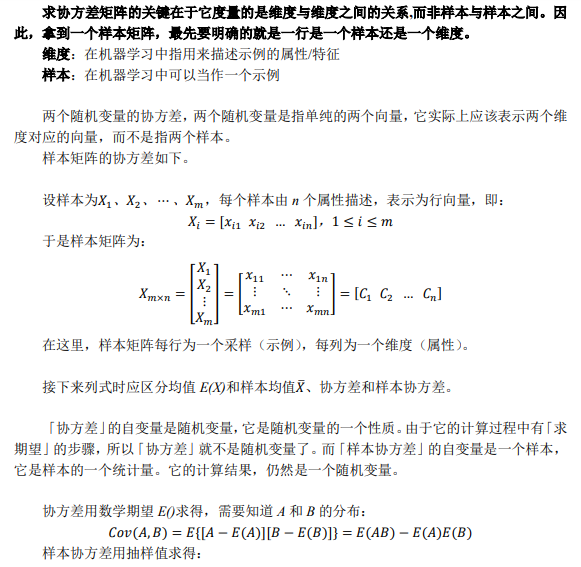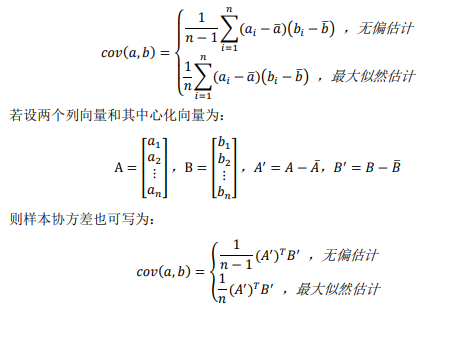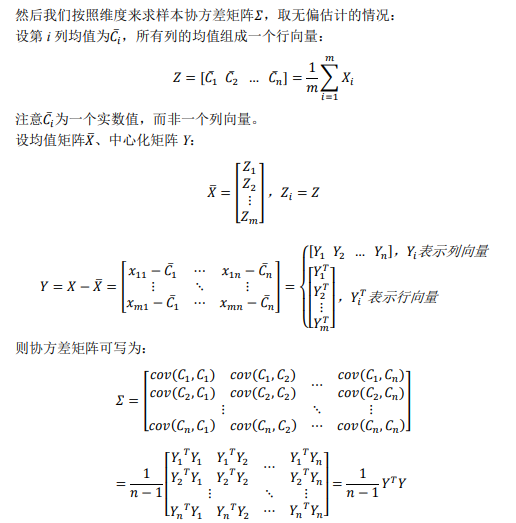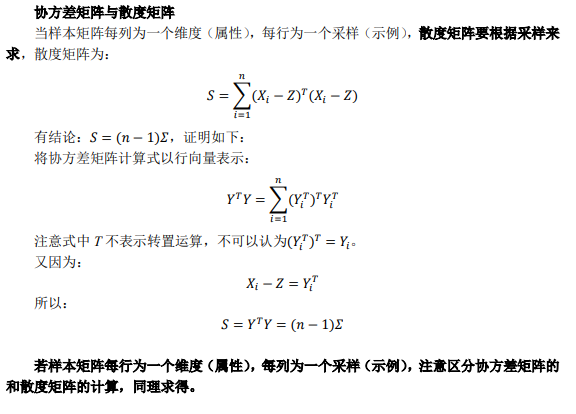展开全文• 对于一般的分布，直接代入E(X)之类的就可以计算出来了，但真给你一个具体数值的分布，要计算协方差矩阵，根据这个公式来计算，还真不容易反应过来。网上值得参考的资料也不多，这里用一个例子说明协方差矩阵是怎么...
• 上一次我们学习了PCA的过程，并且在最后还特意为大家介绍了协方差矩阵以及协方差矩阵的特征值和特征向量的作用。 Now , Review it Together… 1：协方差 2：协方差矩阵 换算成矩阵运算，假设矩阵A是M行N列，每一列...机器学习 线性代数
• ## 协方差矩阵详解

千次阅读 2014-10-18 14:17:39
这样，我们就得到了计算协方差矩阵所需要的所有数据，可以调用Matlab的cov函数直接得到协方差矩阵： 图 5 使用Matlab的cov函数直接计算样本的协方差矩阵 计算的结果，和之前的数据填入矩阵后的结果...协方差
• 那么x的协方差矩阵为Y = [var(x1,x1) var(x1,x2) ; var(x2,x1) var(x2,x2)];此协方差矩阵是一个正定的对称矩阵，var是方差的matlab求解指令；可以看出，协方差矩阵的对角线为每一维信号的方差，非对角线元素为两个...
• 其二, 按照大学的线性数学水平来理解, 它比较复杂一点,可以看做是两组数据的向量夹角的余弦. 皮尔逊相关的约束条件 从以上解释, 也可以理解皮尔逊相关的约束条件: 1 两个变量间有线性关系 2 变量
• 2）评估两组数据之间的互相关联程度，大于0正相关，小于0负相关，等于0不相关。 但此种计算方法反映的是“线性相关”程度，对非线性相关结果不可靠（可采用斯皮尔曼等级相关系数(Spearman correlation coefficient)...matlab
• 1.1 , 1.97, -0.31, -0.55, 2.06, -0.24, -1.44, 1.56, 3.69, 0.53, 2.3 , 1.09, -2.63, 0.29, 1.3 , -1.54, 3.19] 每行23个数 协方差矩阵公式就是下面的写法 上一步对两组数据求协方差介绍了步骤，协方差矩阵就是...python 算法
• 解释一下，主函数主要是设定好已知的样本数目和均值和协方差矩阵。然后调用multivrandn就可以了，详细解释一下nestfunction，nestfunction里面有chol这个函数，这个函数就是乔木斯分解，数值分析里有讲过。它的作用...机器学习
• 这样，我们就得到了计算协方差矩阵所需要的所有数据，可以调用Matlab的cov函数直接得到协方差矩阵： 图 5 使用Matlab的cov函数直接计算样本的协方差矩阵 计算的结果，和之前的数据填入矩阵后的结果完全相同。 ...
• 根据D（A＋B）＝D（A）＋D（B）+2COV（A，B）计算 private double GetConv(double[] a, double[] b) { if (a.Length != b.Length) { return 0;//error }
• 前言我们在分析两组变量之间的相关性时，比如X=[X1,X2,...,Xm]X=[X_1,X_2,...,X_m]和Y=[Y1,Y2,...,Yn]Y=[Y_1,Y_2,...,Y_n],最原始的方法就是直接计算X和Y的协方差矩阵，矩阵有m*n个值。有了协方差矩阵就得到了两两...机器学习 统计学 CCA 典型相关分析
• ## 协方差计算方法

千次阅读 2015-09-28 21:28:51
关于协方差矩阵，对于抽象的公式一时不能正确的反应和理解，看到ybdesire的有关介绍，恍然大悟。在这里表示感谢。   协方差的定义   对于一般的分布，直接代入E(X)之类的就可以计算出来了，但真给你一个具体...
• ## 协方差的计算方法

千次阅读 2019-11-19 22:12:21
对于一般的分布，直接代入E(X)之类的就可以计算出来了，但真给你一个具体数值的分布，要计算协方差矩阵，根据这个公式来计算，还真不容易反应过来。网上值得参考的资料也不多，这里用一个例子...统计学习
• ## 矩阵的理解

千次阅读 2016-06-25 11:32:54
这样的话就可以用向量和矩阵来表示线性空间和线性变换，同其他的数学形式一样，矩阵是一种表达形式（notation），而这一方面可以简洁地表达出我们平时遇到的如线性方程和协方差关系的协方差矩阵等，另一方面又给...
• 以多维几何空间考虑，两组向量的相似度可以描述为在多维几何空间中的距离关系，距离越远，相似度越低。对原文有修改，如有疑惑，请拜访原文。 原文地址：距离 计算 总结 在做分类时常常需要估算不同样本之间的...
• 目录协方差 cov(x)- x 为一个样本向量- x 为一个样本矩阵协方差 cov(x,y)变异系数 c.v 首先看看 均值，样本方差，样本协方差 公式区别 其中样本方差公式中为什么除的n-1而不是n，样本协方差同样除的是n-1而不是n...协方差 变异系数
• 这样的话就可以用向量和矩阵来表示线性空间和线性变换，同其他的数学形式一样，矩阵是一种表达形式（notation），而这一方面可以简洁地表达出我们平时遇到的如线性方程和协方差关系的协方差矩阵等，另一方面又给...
• 当然书中主要是针对有限维的情况来讨论的，这样的话就可以用向量矩阵来表示线性空间和线性变换，同其他的数学形式一样，矩阵是一种表达形式（notation）， 而这一方面可以简洁地表达出我们平时遇到的如线性数学
• 在NLP领域中，为了能表示人类的语言符号，一般会把这些符号转成一种数学向量形式以方便处理，我们把语言单词嵌入到向量空间中就叫词嵌入（word embedding）。 比如有比较流行的谷歌开源的 word2vec ，它能生成词...机器学习 NLP 典型相关分析 CCA
• 一、相关系数第一次理解 概念 ：Pearson相关系数 （Pearson CorrelationCoefficient）是用来衡量两个数据集合是否在一条线上面，它用来衡量 ...X、Y的协方差矩阵，由X、Y的方差和协方差组成：相关系数...